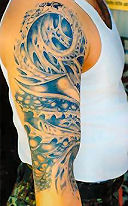Search IntMath
Close

# Fractal tattoos

By Murray Bourne, 28 Jan 2006How cool is this? Mathematical tattoos.

For the mathematics behind this, see fractals.

I might get one of these on my left nipple.

Juz kidding...

### Comment Preview

HTML: You can use simple tags like <b>, <a href="...">, etc.

To enter math, you can can either:

1. Use simple calculator-like input in the following format (surround your math in backticks, or qq on tablet or phone):
a^2 = sqrt(b^2 + c^2)
(See more on ASCIIMath syntax); or
2. Use simple LaTeX in the following format. Surround your math with $$ and $$.
$$\int g dx = \sqrt{\frac{a}{b}}$$
(This is standard simple LaTeX.)

NOTE: You can mix both types of math entry in your comment.